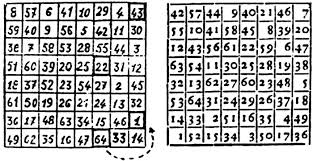# No knight's tourIt is well-known that there is a knight's tour on an $8\times 8$ chessboard; that is, a path of knight moves starting and ending at the same point which touches each other square exactly once along the way. Let's think about knight's tours on other rectangular chessboards.

(a) Consider the following question: how many knights can you place on an $m \times n$ chessboard such that no two knights attack each other? Show that the answer is at least $mn/2$.

(b) Show that if there is a knight's tour on an $m \times n$ chessboard, then $mn$ is even.

(c) Suppose there is a knight's tour on an $m \times n$ chessboard. Show that there are exactly two ways to place $mn/2$ knights on the board so that no two attack each other. (The "exactly" is important here!)

(d) Show that there is no knight's tour on a $4 \times n$ chessboard. (Hint: use part (c).)Note by Patrick Corn
6 years, 6 months ago

This discussion board is a place to discuss our Daily Challenges and the math and science related to those challenges. Explanations are more than just a solution — they should explain the steps and thinking strategies that you used to obtain the solution. Comments should further the discussion of math and science.

When posting on Brilliant:

• Use the emojis to react to an explanation, whether you're congratulating a job well done , or just really confused .
• Ask specific questions about the challenge or the steps in somebody's explanation. Well-posed questions can add a lot to the discussion, but posting "I don't understand!" doesn't help anyone.
• Try to contribute something new to the discussion, whether it is an extension, generalization or other idea related to the challenge.

MarkdownAppears as
*italics* or _italics_ italics
**bold** or __bold__ bold
- bulleted- list
• bulleted
• list
1. numbered2. list
1. numbered
2. list
Note: you must add a full line of space before and after lists for them to show up correctly
paragraph 1paragraph 2

paragraph 1

paragraph 2

[example link](https://brilliant.org)example link
> This is a quote
This is a quote
    # I indented these lines
# 4 spaces, and now they show
# up as a code block.

print "hello world"
# I indented these lines
# 4 spaces, and now they show
# up as a code block.

print "hello world"
MathAppears as
Remember to wrap math in $$ ... $$ or $ ... $ to ensure proper formatting.
2 \times 3 $2 \times 3$
2^{34} $2^{34}$
a_{i-1} $a_{i-1}$
\frac{2}{3} $\frac{2}{3}$
\sqrt{2} $\sqrt{2}$
\sum_{i=1}^3 $\sum_{i=1}^3$
\sin \theta $\sin \theta$
\boxed{123} $\boxed{123}$

Sort by:

No one has tried any of these. So I will mention a hint that helps with many of these questions: if two knights are on the same color square, they cannot attack each other.

- 6 years, 6 months ago

That directly proves (a).

Knights swap colours every time they move. That proves (b). (I think.)

- 5 years, 6 months ago

Yep, keep going!

- 5 years, 6 months ago

c) From b) is it clear that $mn/2$ is an integer. In particular, there is the same number of black squares as white squares. This proves there is at least two ways (take the set of knights only on white squares; similar for black). Now suppose that there existed another placing of knights. Then at least one knight is white and at least one knight is black. However, this is impossible; because of the existence of the knight's tour, if there were$k$ black knights then at least $k+1$ white squares are attacked, leaving $mn/2-k-1$ white squares left to place knights, not enough to place $mn/2-k$ white knights.

- 5 years, 3 months ago

d) From c) it suffices to show there are more than two ways to place the knights on the $4\times n$ board such that no knight attacks another. In fact, there are at least three: the two standard ways of placing all knights on black/white squares, and the third way of placing knights on the top row of $n$ squares, and placing knights on the bottom row of $n$ squares. This proves the impossibility of a knight's tour.

- 5 years, 3 months ago

There you go. I like this proof a lot better than the other "standard" one with colorings. The steps are all very simple, and at the end you get something nice and nontrivial.

- 5 years, 3 months ago